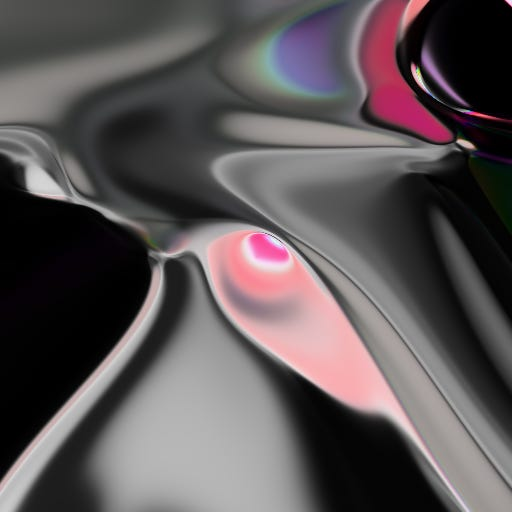# Generate abstract random art with a neural network

Source: Deep Learning on Medium

Recently I saw a post by David Ha titled “Neural Network Generative Art in Javascript” using randomly initialized fully connected neural networks to generate images of a desired size. Since I am very interested into art and generative models I wanted to implement this algorithm in python.

I assume the readers are familiar with neural networks and understand computing a forward pass and the backpropagation, although for this algorithm only the forward pass is needed. For a quick intro have a look at this medium blogpost about neural nets.

As deep neural networks have become very popular as classification or regression models those models can also be used as generative models to create artificial data such as new images or even text data as seen in following examples : (1) generate art from a genre using VAE or DCGAN , (2) generate text using recurrent neural networks .

Below are some generated images done with the abstract art generation algorithm: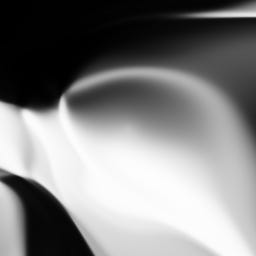Generated Black White Image (256×256)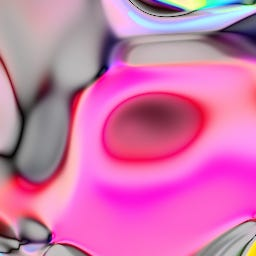Generated HSL Image (256×256)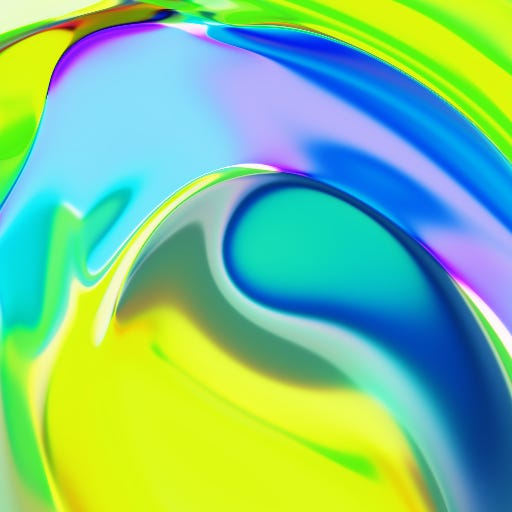Generated RGB Image (512×512)

The entire project can be found on my github repo neural-net-random-art. If you want to understand the algorithm in depth have a look at the jupyter notebook. For just creating images it is recommended to use the python main program by executing it on the shell.

In contrast to the classification/regression settings this algorithm does not need valid training data, meaning to have input features X and labels y. What the algorithm actually just does is the initialization step of the weight matrices W_i of a dense network and computing image_height*image_width forward steps with the randomized weights. One can think of computing several activations through each hidden layer and passing those forward to the output layer which consists of 3 output neurons for R, G, B value. For the output neurons the sigmoid activation function will be used in order to guarantee RGB values in range 0 and 1. As an additional feature the alpha channel will be modelled. Note that if we do not want alpha channel modelling this value will always be 1. So for the image we implement a 4-dimensional numpy array, where the first 3 dimensions are R,G,B and the fourth channel is the alpha channel:

#### Neural Network Class

For the neural network we only need the forward pass calculation and hence define a `NeuralNet` class which consists of all methods needed (note that class methods like computation of gradients are backpropagation are not implemented):

Important are the following lines:

1. Initialization of weight matrices and biases in line 9–11 using a standard normal distribution N(0,1)
2. Computation of forward pass in line 67–72 where the weighted sum will be computed using two class methods: First the weighted sum of the input features / output previous hidden layer will be computed with the current hidden layer and then this result will be added with the bias term (weight). After this computation the weigted sum will be transformed via an activation function and saved in the `self.out` list.

#### Neural Network Architecture

For this art generation process our neural network will have an input layer of 5 neurons, enhanced in one vector. We obtain input= (x, y, r, z1, z2). From the input layer through all the hidden layers the activations will be computed. It is recommended to use `tanh` activation function since this maps the output into range -1 and 1. For the input vector one can say that those values will always be small values either between -0.5 and 0.5 (for x,y,r) or -1 and 1 (for z1,z2). The explanation will be made later below. The output layer consists in general of 3 neurons (and 4 if the alpha channel should be modelled as well). Those neurons should represent R,G,B,(A) values. Since we want to get float RGBA / color values we use as last activation function in the output layer the `sigmoid` function since this maps into range 0 and 1.

The algorithm also allows to have different colormodes so besided RGB there is CMYK or HSL and HSV.

The code block below is a function to get the neural network architecture parameters to pass to the `NeuralNet` class:

An example network would be if executing following lines:

Leading to following neural network: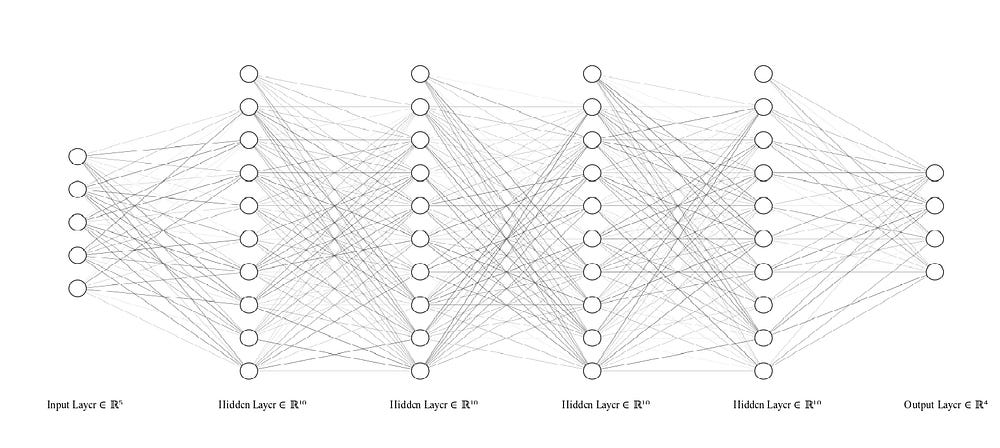Example neural network architecture

#### Generating the image

For the image generation one can think of populating a 2D matrix with a RGB value for each row and column from the output of our neural network. Hence the computation will be done in a 2-nested for loop. Here’s the code for populating the image, note that the image is actually 4-dimensional numpy array:

For the input value for each row and column one can see that the x and y value will be in range -0.5 and 0.5 and due to the nested for loop we can think of x and y as linear functions of the iterator `i`and `j` . The radius r is the computed value of a circle equation. If you want to add more correlation you can think of other mathemical shapes such as triangle, parabolic or square root function for example. If you want to add them, just make sure you increase the number of input neurons in line 2 of `prep_nnet_arch.py`. The z1 and z2 values are just random values between -1 and +1. In line 17–20 the population of the image with the RGBA values will be done. Note that thouse `r, g, b, a` values are actually outputs from the neural network. The `get_color_at()` function is just a wrapper for it doing several other steps. For detailed version have a look at the full script.

Feel free to change the script and try out different network architectures and see how the generated images change. I’d like to hear some feedback. Either here on medium, via mail to tuanle@hotmail.de or on https://www.linkedin.com/in/tuan-le618/ .